# How to convert Column Letter to Number in Excel

This post will guide you how to convert column letter to number in Excel. How do I convert letter to number with a formula in Excel. How to convert column letter to number with VBA macro in Excel. How to use a User Defined Function to convert letter to number in Excel.

## Convert Column Letter to Number with a Formula

If you want to convert a column letter to a regular number, and you can use a formula based on the COLUMN function and the INDIRECT function to achieve the result.

For example, you need to convert a column letter in Cell B1 to number

Just like this:

`=COLUMN(INDIRECT(B1&1))`

Type this formula into a blank cell such as: C1, and press Enter key, and then drag the AutoFill Handle over to other cells to apply this formula.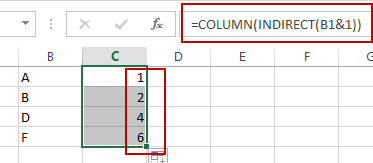The INDIRECT function will convert the text into a proper Excel reference and then pass the result to Column function to get the column number for the reference.

## Convert Column Letter to Number with VBA Macro

You can also use an Excel VBA Macro to achieve the result of converting column letter into its corresponding numeric value. Just do the following steps:

#1 open your excel workbook and then click on “Visual Basic” command under DEVELOPER Tab, or just press “ALT+F11” shortcut.#2 then the “Visual Basic Editor” window will appear.

#3 click “Insert” ->”Module” to create a new module.#4 paste the below VBA code into the code window. Then clicking “Save” button.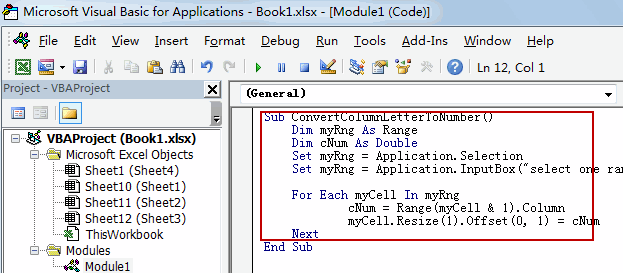```Sub ConvertColumnLetterToNumber()
Dim myRng As Range
Dim cNum As Double
Set myRng = Application.Selection
Set myRng = Application.InputBox("select one range that contain column letters", "ConvertColumnLetterToNumber", myRng.Address, Type:=8)

For Each myCell In myRng
cNum = Range(myCell & 1).Column
myCell.Resize(1).Offset(0, 1) = cNum
Next
End Sub```

#5 back to the current worksheet, then run the above excel macro. Click Run button.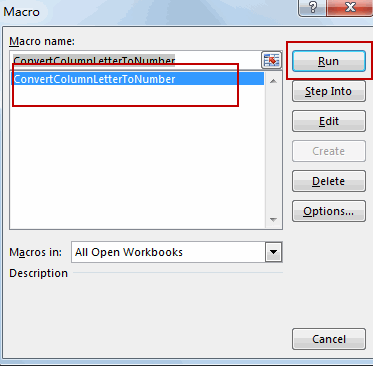#6 select one range that contain column letters, such as B1:B4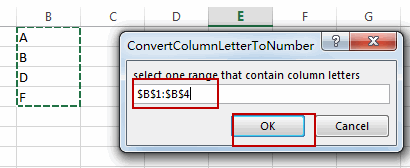#7 let’s see the result: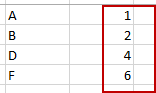## Convert Column Letter to Number with User Defined Function

Excel does not have a built in formula to convert column letter to a numeric number, but you can write down a User Defined Function to achieve the result. Just do the following steps:

#1 repeat the above step 1-3

#2 paste the below VBA code into the code window. Then clicking “Save” button.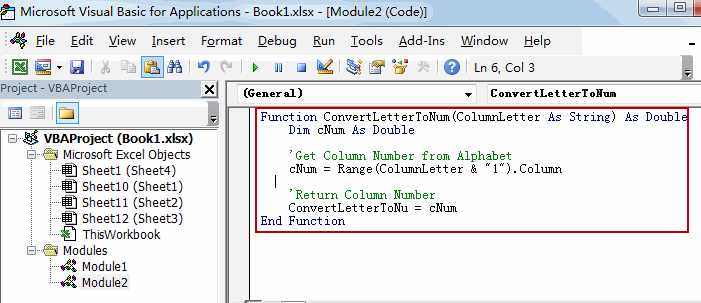```Function ConvertLetterToNum(ColumnLetter As String) As Double
Dim cNum As Double

'Get Column Number from Alphabet
cNum = Range(ColumnLetter & "1").Column

'Return Column Number
ConvertLetterToNum = cNum
End Function```

#3 back to the current worksheet, then type the following formula in a blank cell. press Enter key.

=ConvertLetterToNum(B1)

#4 select the cell C1, and  drag the AutoFill Handle over to other cells to apply this formula.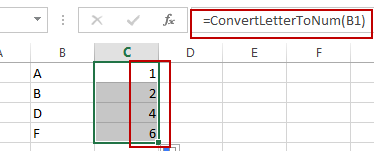## Convert Number to Column Letter

If you want to convert column number to an Excel Column letter, you can use another formula based on the SUBSTITUTE function and the ADDRESS function. Just like this:

`=SUBSTITUTE(ADDRESS(1,C1,4),"1","")`

Type this formula into Cell D1, and press Enter key. And then drag the AutoFill handle over to other cells to apply this formula.

Let’s see the last result: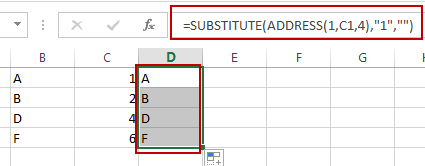Video: Convert Column Letter to Number

### Related Functions

• Excel Substitute function
The Excel SUBSTITUTE function replaces a new text string for an old text string in a text string.The syntax of the SUBSTITUTE function is as below:= SUBSTITUTE  (text, old_text, new_text,[instance_num])….